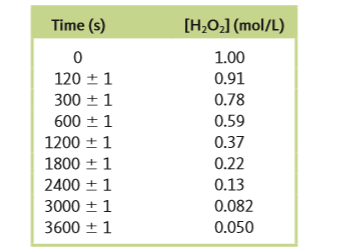# Problem: The decomposition of hydrogen peroxide was studied, and the following data were obtained at a particular temperature:Assuming thatRate = - Δ [H2O2] / Δtdetermine the rate law, the integrated rate law, and the value of the rate constant. Calculate [H2O2] at 4000. s after the start of the reaction.

⚠️Our tutors found the solution shown to be helpful for the problem you're searching for. We don't have the exact solution yet.

###### Problem Details

The decomposition of hydrogen peroxide was studied, and the following data were obtained at a particular temperature:Assuming that

Rate = - Δ [H2O2] / Δt

determine the rate law, the integrated rate law, and the value of the rate constant. Calculate [H2O2] at 4000. s after the start of the reaction.